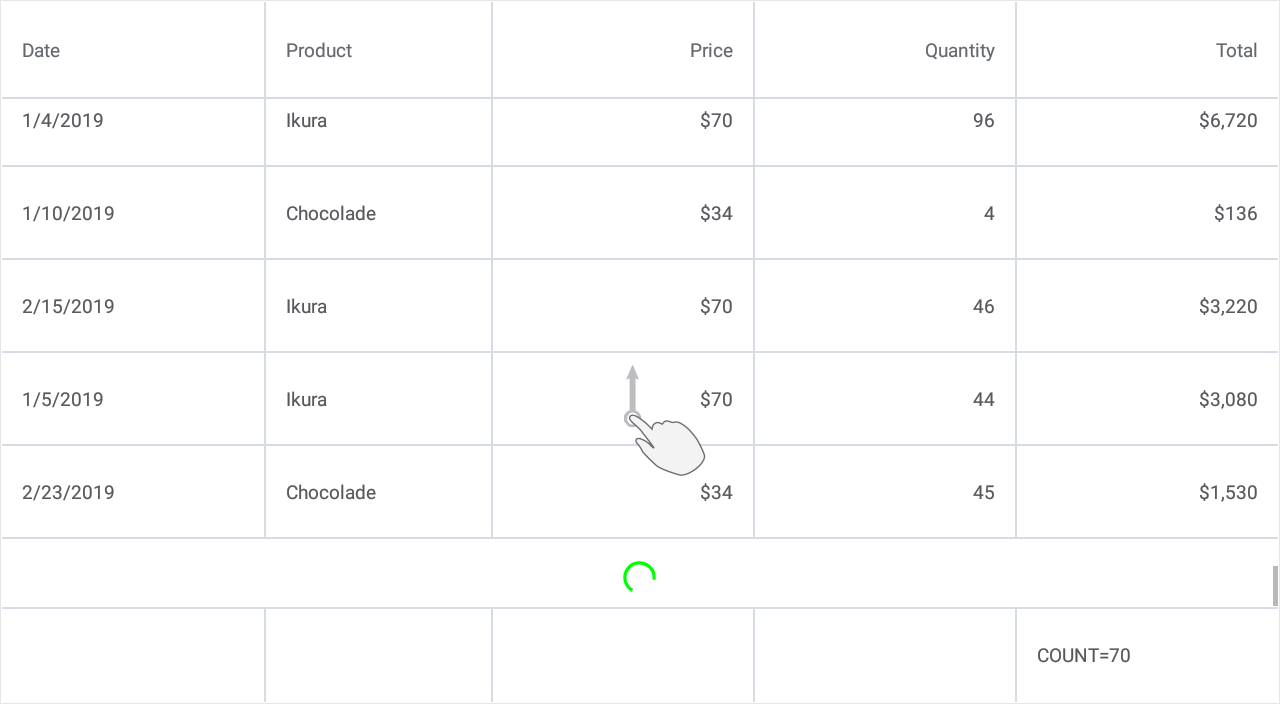This example shows how to implement the grid’s load-more functionality - when a user scrolls to the bottom of the grid, a set of new data items is added to the end of the grid.

1. Set the DataGridView.IsLoadMoreEnabled property to true to enable the grid’s load-more functionality.
2. Create a command to be executed when a user scrolls to the bottom of the grid. Set the DataGridView.IsRefreshing property to false after data is loaded to hide the loading indicator in the grid.

3. Bind the DataGridView.LoadMoreCommand property to the created command.

In this example, data items for each next load (ten new orders) are generated randomly in code. The maximum number of loads a user is allowed to perform is 3. The total summary displays the count of data items currently loaded to the grid (it is automatically updated after each load).``````using System;
using System.Collections.Generic;
using System.Collections.ObjectModel;

public class Product {
string name;
int unitPrice;

public Product(string name, int unitPrice) {
this.name = name;
this.unitPrice = unitPrice;
}
public string Name {
get { return name; }
set { name = value; }
}
public int UnitPrice {
get { return unitPrice; }
set { unitPrice = value; }
}
}

public class Order {
DateTime date;
Product product;
int quantity;

public Order() {
this.date = DateTime.Today;
this.product = new Product("", 0);
this.Quantity = 0;
}
public Order(DateTime date, Product product, int quantity) {
this.date = date;
this.product = product;
this.quantity = quantity;
}
public DateTime Date {
get { return date; }
set { date = value; }
}
public Product Product {
get { return product; }
set { product = value; }
}
public int Quantity {
get { return quantity; }
set { quantity = value; }
}
}

public class OrderData {
const int orderCount = 50;
public ObservableCollection<Order> Orders { get; set; }

public OrderData() {
random = new Random((int)DateTime.Now.Ticks);
products = new List<Product>();
GenerateProducts();
Orders = new ObservableCollection<Order>();
for (int i = 0; i < orderCount; i++)
}

for (int i = 0; i < 10; i++)
}

Order GenerateOrder() {
Order order = new Order(new DateTime(2020, 1, 1).AddDays(random.Next(0, 60)),
RandomItem<Product>(products), random.Next(1, 100));
return order;
}

T RandomItem<T>(IList<T> list) {
int index = (int)(random.NextDouble() * 0.99 * (list.Count));
return list[index];
}

void GenerateProducts() {